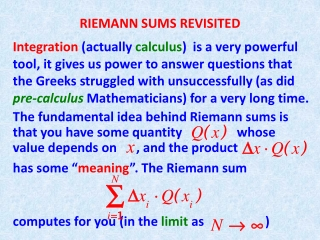DownloadDownload PresentationRIEMANN SUMS REVISITED

# RIEMANN SUMS REVISITED

Download Presentation## RIEMANN SUMS REVISITED

- - - - - - - - - - - - - - - - - - - - - - - - - - - E N D - - - - - - - - - - - - - - - - - - - - - - - - - - -
##### Presentation Transcript

1. RIEMANN SUMS REVISITED Integration (actually calculus) is a very powerful tool, it gives us power to answer questions that the Greeks struggled with unsuccessfully (as did pre-calculus Mathematicians) for a very long time. The fundamental idea behind Riemann sums is that you have some quantity whose value depends on , and the product has some “meaning”. The Riemann sum computes for you (in the limit as )

2. … the total amount of the “meaning” . Here are a couple of examples: 1. Height base area 2. Flow through time total amount (gals) a valve (gal/sec) through valve The next example is better illustrated through a figure. Think of a solid in space (an egg in the figure) and think you can slice the egg with parallel slices. Assume that the slices are nearly cylindrical, perhaps with a funny shaped bases, but ….

3. … you know the area of the base of the slice at position . Then the Riemann sum is the sum of the volumes of the (nearly) cylin-drical slices. As the slices get thinner, (and more of them) the limit of the Riemann sums (remember, it’s the integral !) gives us the volume of the egg. Here is the figure

4. (You are looking at the football from below) The nice thing about the figure is that we can actually compute the area of the violet circles. In general this may not be so, as in the

5. following figure (borrowed from Prof. Pilkington) Who knows what the areas of those cross-sections are!

6. There is however one particular case when those cross-section areas are computable, namely in the case of SOLIDS OF REVOLUTION. These are solids which are obtained by rotating the graph of some function . The resulting surface bounds a solid whose cross-sectional areas are circles, area = . Therefore the volume of the solid is computed as Let’s do some examples. We will rediscover …

7. … the formulas for the volumes of a sphere, a cylinder, a cone. Then we will find the volume of that football, and some more interesting solids. In all examples you will see that the trick is to realize which function one needs to rotate about which line. We get: Sphere ------- Volume = Cylinder ----- Volume = Cone --------- Volume =

8. For each of the three solids shown we try to determine which region we rotate about which line. We have four choices, namely

9. Rotate shaded area about y-axis In both rotations the left-hand approach is easier (why?… look at the equation of the line …)

10. One can rotate either a quarter circle or a semi-circle, about either axes, as shown

13. We’ll recover the formula for the volume of a cone. We will rotate about the x-axis.

14. (see figure) The formula is: (Check it out!)

15. We promised the volume of a football. We will rotate the curve (shown in the figure) about the x-axis. We have (see figure)

16. We obtain the formula: (Check it out !) Note the lack of symmetry in and . !

17. We conclude with a final REMARK. • Any area can be considered as between two curves. (The lines and are indeed curves !) • When rotating about the x-axis the equations MUST be • When rotating about the y-axis the equationsMUST be

18. One last example, about as hard as I can concoct! Look at the pink-shaded area shown in the figure: The two equations (color coded) are: and . We rotate the area about the y-axis, then about the x-axis.

19. We will compute the volume of both solids generated. First about the y-axis. Recall the REMARK, we must have Unfortunately the right-hand boundary of the figure consists of two “curves”, and What about the limits and ? These are supposed to be limits for . Looking at the figure we conclude that the volume of the solid of revolution obtained when rotating about

20. the y-axis is computed by the two integrals: Which give the answer as (check it out !) Next we rotate about the x-axis. We MUST get

21. This is easier. A glance at the figure shows that the answer is obtained from the integral: which gives Here are the two solids

22. About the y-axis (a round-bottom cylinder with an ice-cream cone carved out.) About the x-axis, (a paraboloid with a smaller ice-cream cone carved out.)

23. Now you should do lots of examplesfrom the textbook, keeping in mind the REMARK. It can be summarized in the statement “Integrate in the variable whose axis you are rotating about.”